# Mark Hampton

•### G-SRT.A2 (2)Are two triangles similar using transformations?

Activity

Mark Hampton

•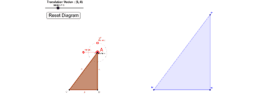### G-SRT.A2 (1)Are two triangles similar using transformations?

Activity

Mark Hampton

•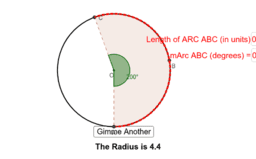### Circle - Find the length and measure of an arc

Activity

Mark Hampton

•### Equations of circles

Activity

Mark Hampton

•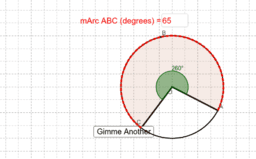### Given a central angle, find measure of arc

Activity

Mark Hampton

•### Circles - Equation of a circle

Book

Mark Hampton

•### Practice drawing a circle that matches an equations

Activity

Mark Hampton

•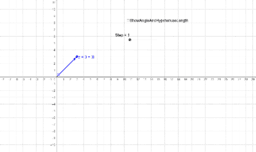### Complex Number Explorer

Activity

Mark Hampton

•### Basic Construction Tools

Activity

Mark Hampton

•### Circle- Angles Inside and Outside

Activity

Mark Hampton

•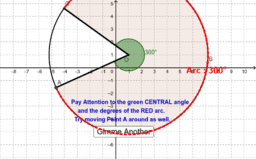### Circle - Central Angle and Intercepted Arc

Activity

Mark Hampton

•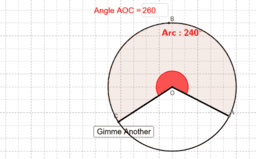### Given the arc size, find the central angle measure

Activity

Mark Hampton

•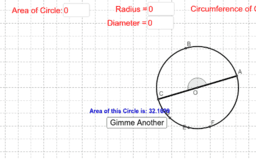### Given area of a circle, find radius, diameter, and circumfer

Activity

Mark Hampton

•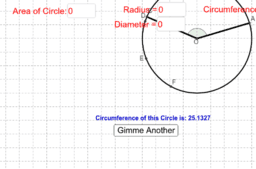### Given Circumference, Find radius, diam, and area

Activity

Mark Hampton

•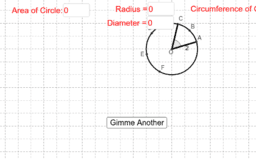### Circles - Given the radius, find the Area, Circ, Diam

Activity

Mark Hampton

•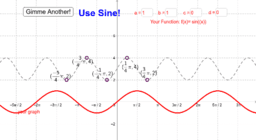### Match the Trigonometric Graph

Activity

Mark Hampton

•### Basic Sine, Cosine, and Tangent Introduction

Activity

Mark Hampton

•### Geometry - Transformations for similarity and congruence

Book

Mark Hampton

•### G-SRT.A2 (3)Are two triangles similar using transformations?

Activity

Mark Hampton

•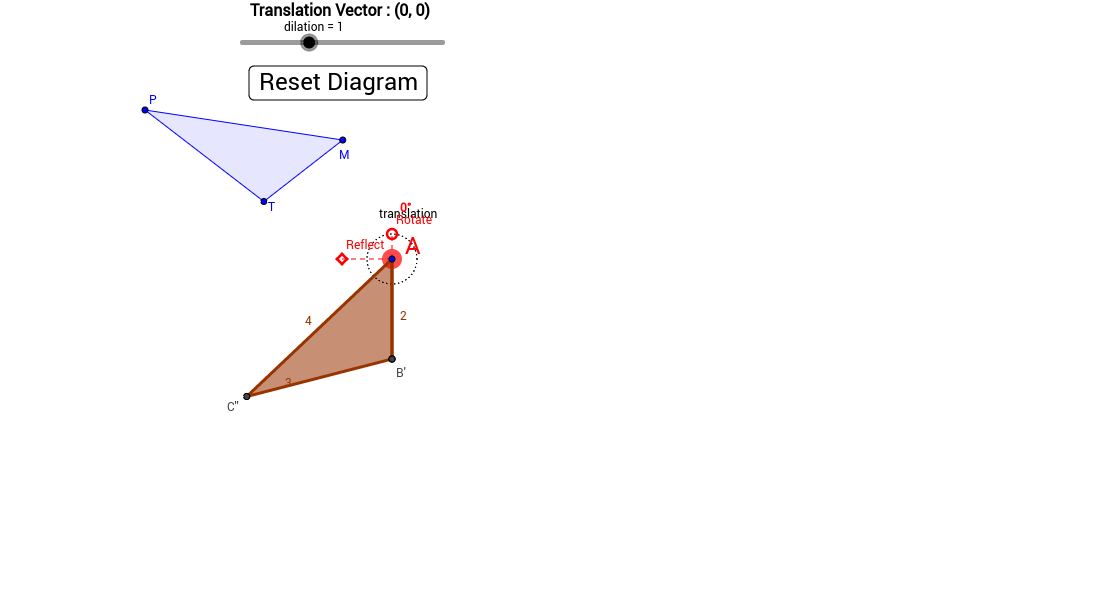### G-SRT.A2 (7)Are two triangles similar using transformations?

Activity

Mark Hampton

•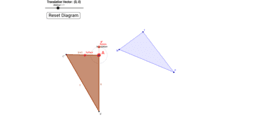### G-SRT.A2 (6)Are two triangles similar using transformations?

Activity

Mark Hampton

•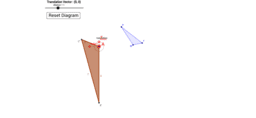### G-SRT.A2 (5)Are two triangles similar using transformations?

Activity

Mark Hampton

•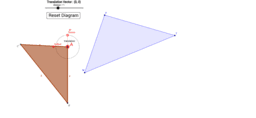### G-SRT.A2 (4)Are two triangles similar using transformations?

Activity

Mark Hampton

•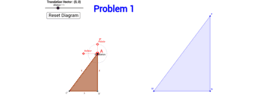Activity

Mark Hampton